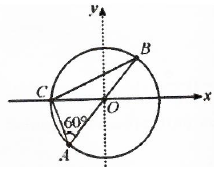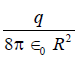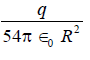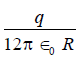Courses

# Consider a system of three charge q/3, q/3 and -2q/3 placed at points A, B and C respectively as shown in figure. Take O to be the circle of radius R and angle CAB = 600.a)The electric field at point O isdirected along the negative x-axis.b)The potential energy of the system is zero.c)The magnitude of the force between the charges at C and B isd)The potential at point O isCorrect answer is option 'C'. Can you explain this answer? Related Test: Electric Potential MCQ Level - 1

## Class 12 Question

By Abhinav Arya · 13 hours ago ·Class 12

## Can you answer this question?

People are searching for an answer to this question.